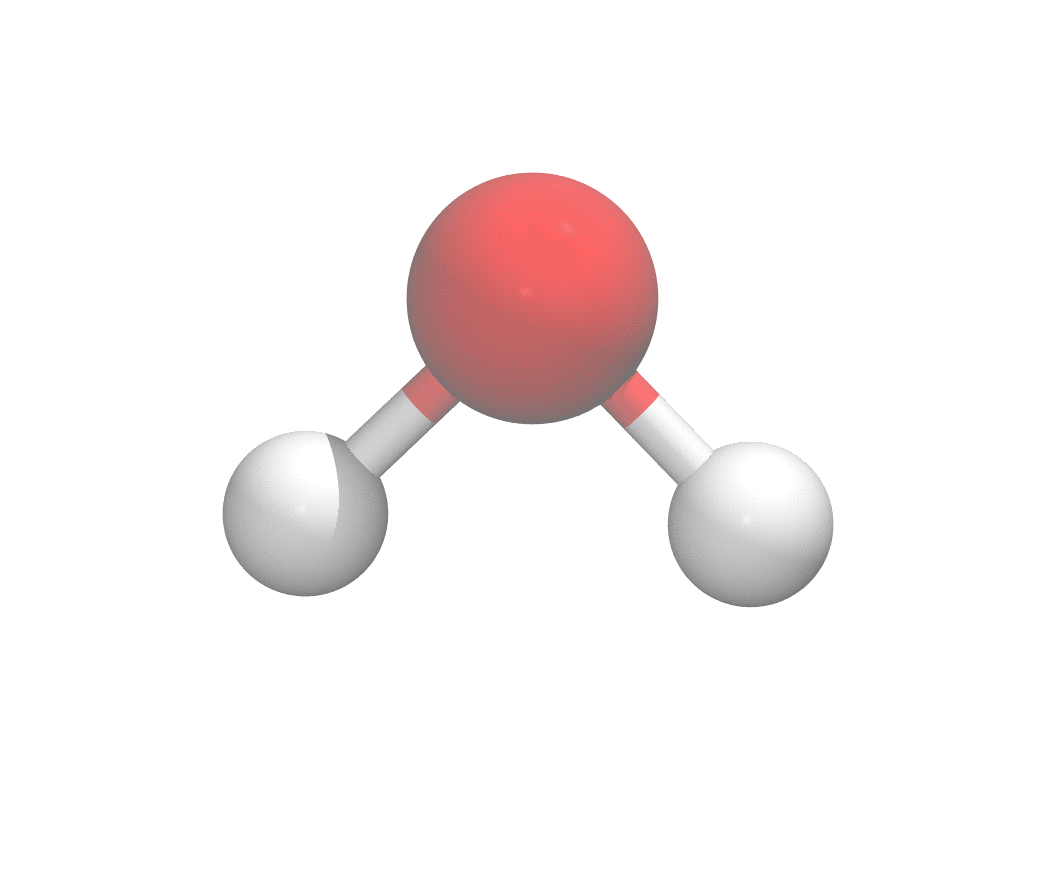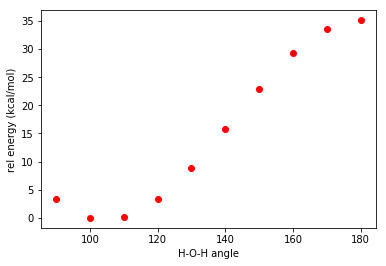# Tutorial 1. Python tutorial for quantum chemistry simulation

### Learning objectives

• Learn basic python scripting to run a quantum chemistry simulation from scratch
• Topics:
1. Geometry generation
2. Input file generation
3. Output file processing

## Prerequisites

1. Conda installed on your system
2. Create new Conda environment called python_tutorial with the following packages installed: jupyter, numpy, matplotlib
3. Terachem installed on a GPU cluster using SGE queue manager

Study the potential energy curve of a water molecule at various H-O-H angles while keep the bond length fixed.## Generate geometries

An .xyz file has the following general format

Put the water molecule on the x-y plane with O at the origin, it’s easy to write down the expression of the coordinates.## TeraChem Input Generation

To run a terachem job on our GPU cluster, one needs at least 3 files: geometry, input deck, submission script.
Here we are going to write a script to generate terachem input deck and submission scripts in batch.
A basic terachem input deck looks like the following:

A basic jobscript for the SGE queueing system looks like the following:

Now let us write a python script that can generate the input file for all the geometries we generated in one batch:

• Create a separate folder for each geometry
• Inside the folder, put in all the needed files: geometry, input deck, and jobscript
• (On the cluster) Job generation and job submission all at once
Basically, the script will create the following tree for us:

First in your terminal, create a new directory. Go to that directory and create a folder called “xyzfiles”, and copy all your generated xyz files into that folder. Run “pwd” and remember the path that is displayed. Then let’s move to that directory in our Jupyter notebook.

And check what you have. Slight modification of your script can enable batch job submission too!

## TeraChem Output Processing

Here we’d like to read out the final energy from the terachem output files and convert the unit to kcal/mol, and plot the potential energy curve.

## Plot results

Here we plot with matplotlib, but there are many tools for creating scientific figures. We will cover that topic in future tutorials.

This will generate the potential energy curve shown at the beginning of this tutorial.

Written on July 22, 2020